Create a formula
Formulas are defined per datasource. To create a formula on a given datasource, open this datasource and select the second tab "Formulas":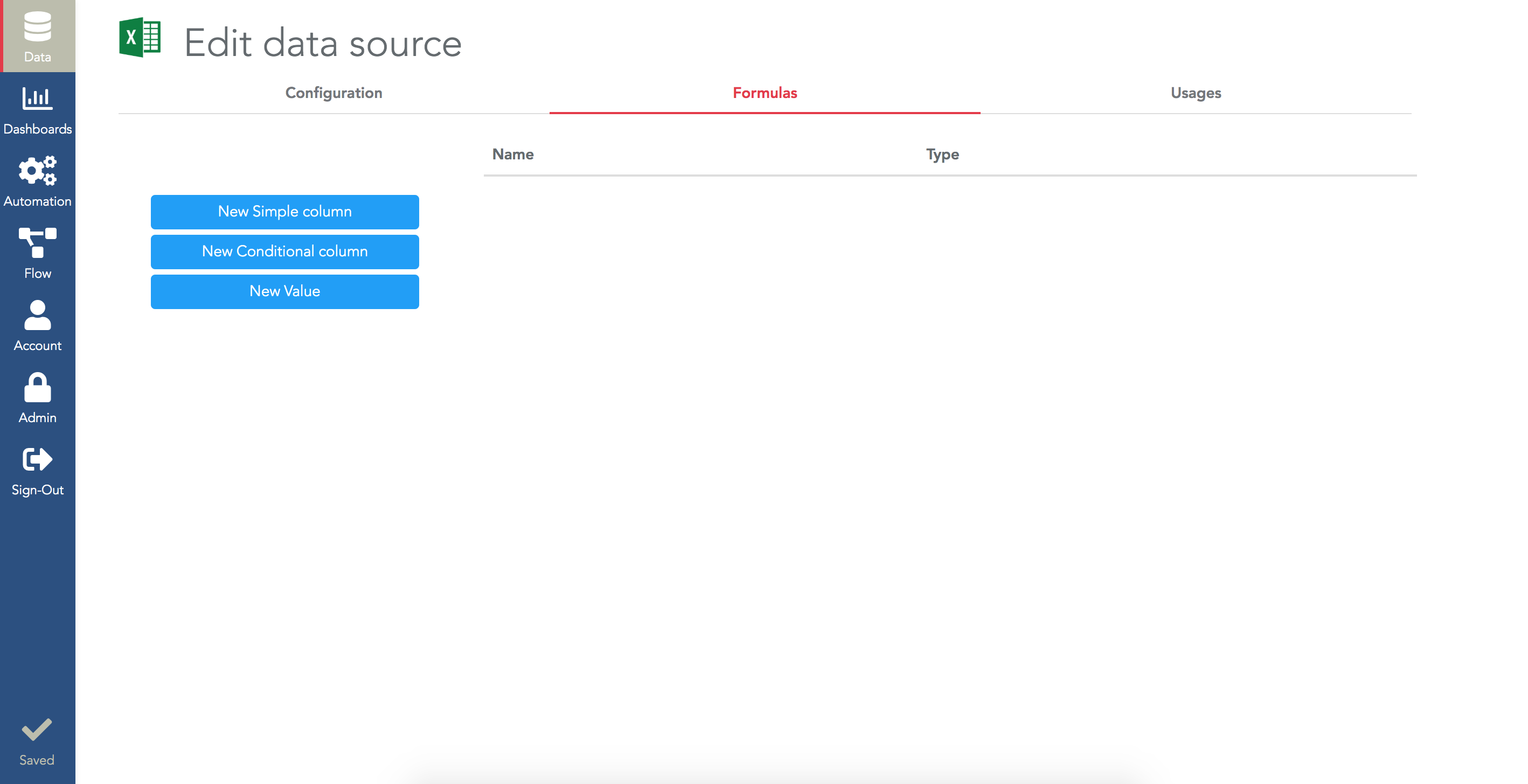There are 3 types of formulas in Serenytics:
• Conditional column: to add a new column to your datasource based on conditions (if/then/else).
• Value: to compute a new single value (e.g. the sum of all the cells of a column).
It's important to understand that a "value" formula is not a new column, it is just a single value. It's dedicated to compute a single KPI.
Create a "simple column" formula
To create such a formula, click on "New Simple column" and enter the content of your formula. For example, if you have a column "Item price" and a column "Nb of items", you can create a column "Basket amount" equal to "[Item price] * [Nb of items]". Columns must be written between square brackets: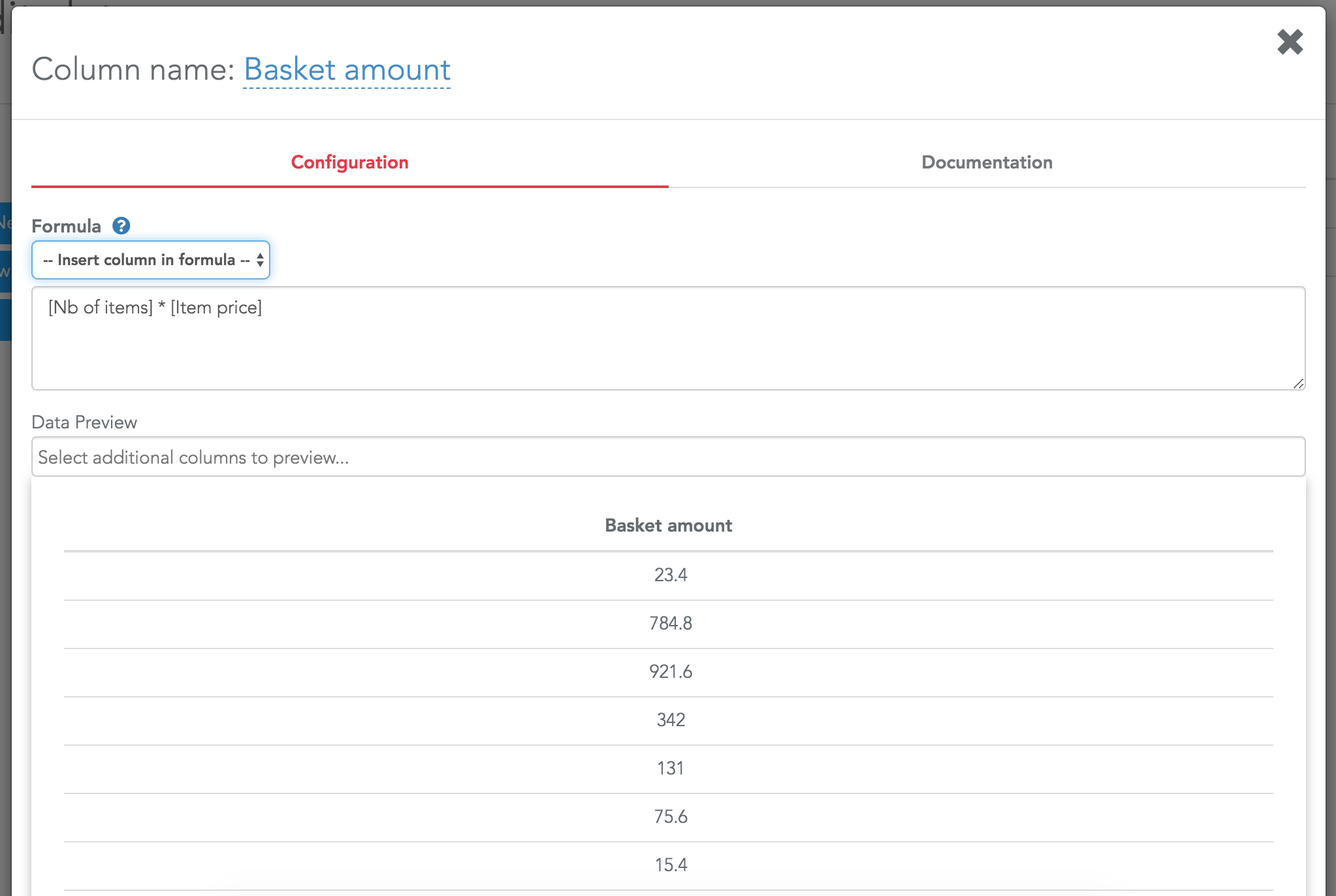Note that instead of typing the names of your columns, you can select them in the field above the formula editor. That will save you from many typos.

Once you have created a new column, you can use it in your widget, there will be no difference between a column that is in the original dataset and a column computed with a formula.

In a formula, there are a lot of Serenytics functions you can use. For example, if you have a date column, you can use the function "extract_date_part" to extract its weekday (i.e. monday, tuesday...): "extract_data_part([MyDateColumn], "weekday")"

The most common functions are:

• extract_date_part
• substring
• like
• datediff
• rank

The full list of functions and their details is available in our documentation.
Create a conditional formula
When you click on "New Conditional" Column, the created formula has a single condition (i.e. an IF statement). As a very simple example, you can compare the amount of the Price column, and write "Big purchase" if the value is above a threshold or "Small purchase" if not: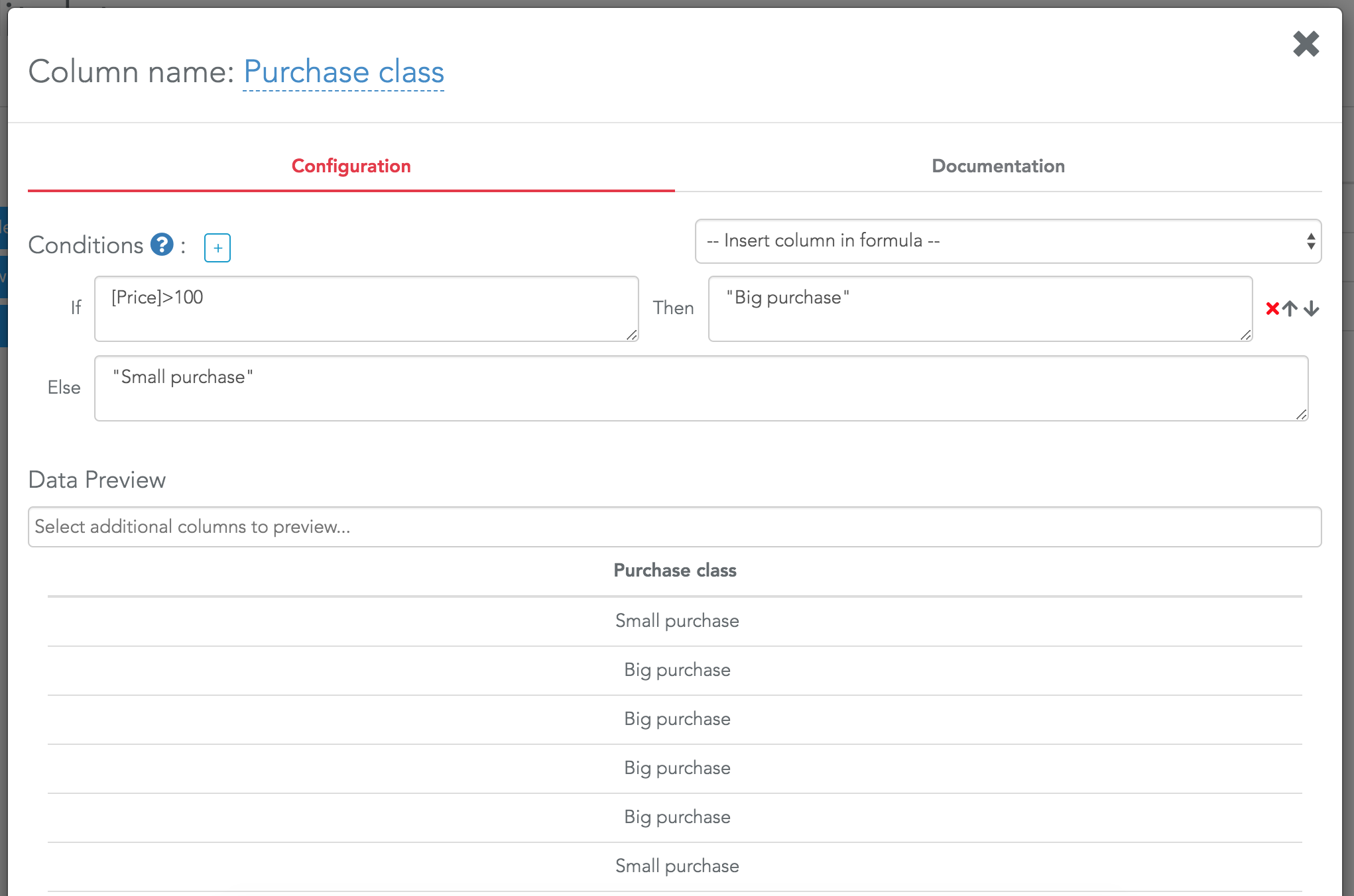Note that any text has to be written between double quotes.

Please keep in mind to name your formula correctly as soon as you create it (the formula above was named "Purchase class").

Using this formula in a dashboard is very straightforward. It appears in all the selectors and you can use it as any other column of your data: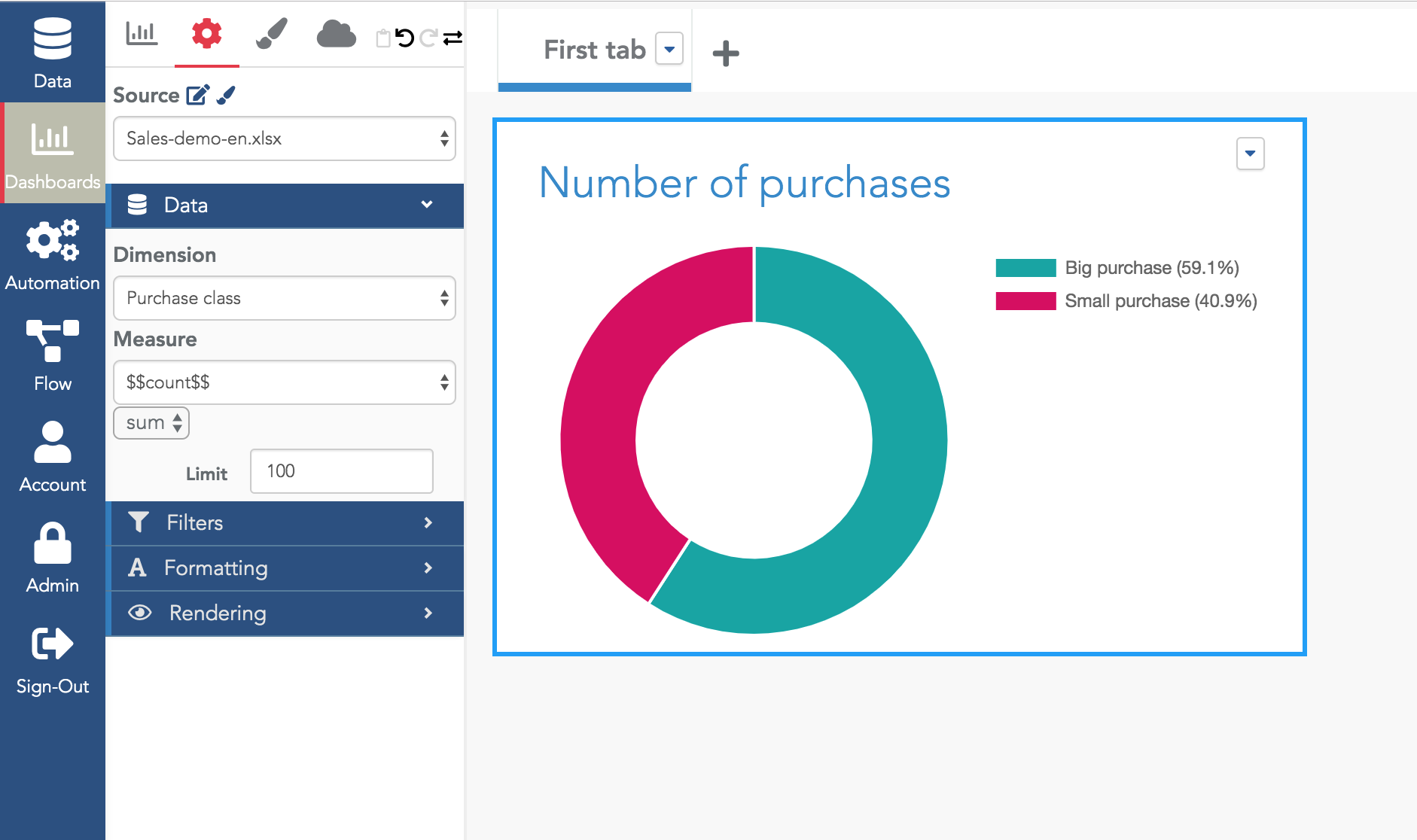To add more condition statements, click on the "+" icon on the right of "Conditions":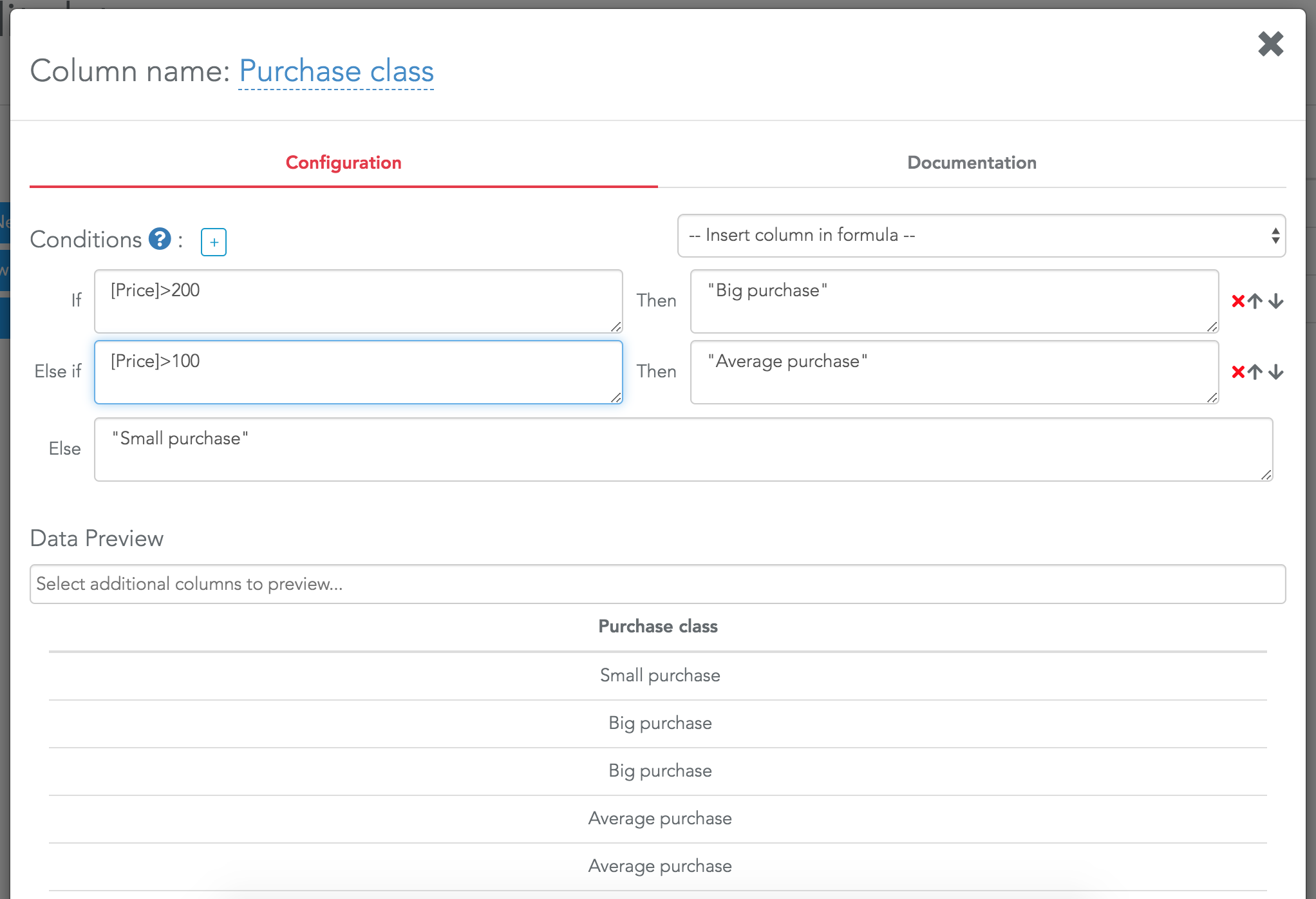Conditions are tested one after another. If a row validates the first condition, it will receive the value of the then statement of the first condition.

Pay attention that if you write:

• IF "[myColumn] > 100" THEN "class 1"
• ELSE IF "[myColumn] > 200" THEN "class 2"
• ELSE "class 3"
No row will never be in class 2 (because if [myColumn]>200, it also means [myColumn] >100).

Note that you can change the order of the conditions and delete them using the icons on the right of the "Then" statements.

When you edit a formula, a preview is automatically displayed at the bottom of the window. You can add other columns to better check the results of your formula by selecting them in the selector above the preview: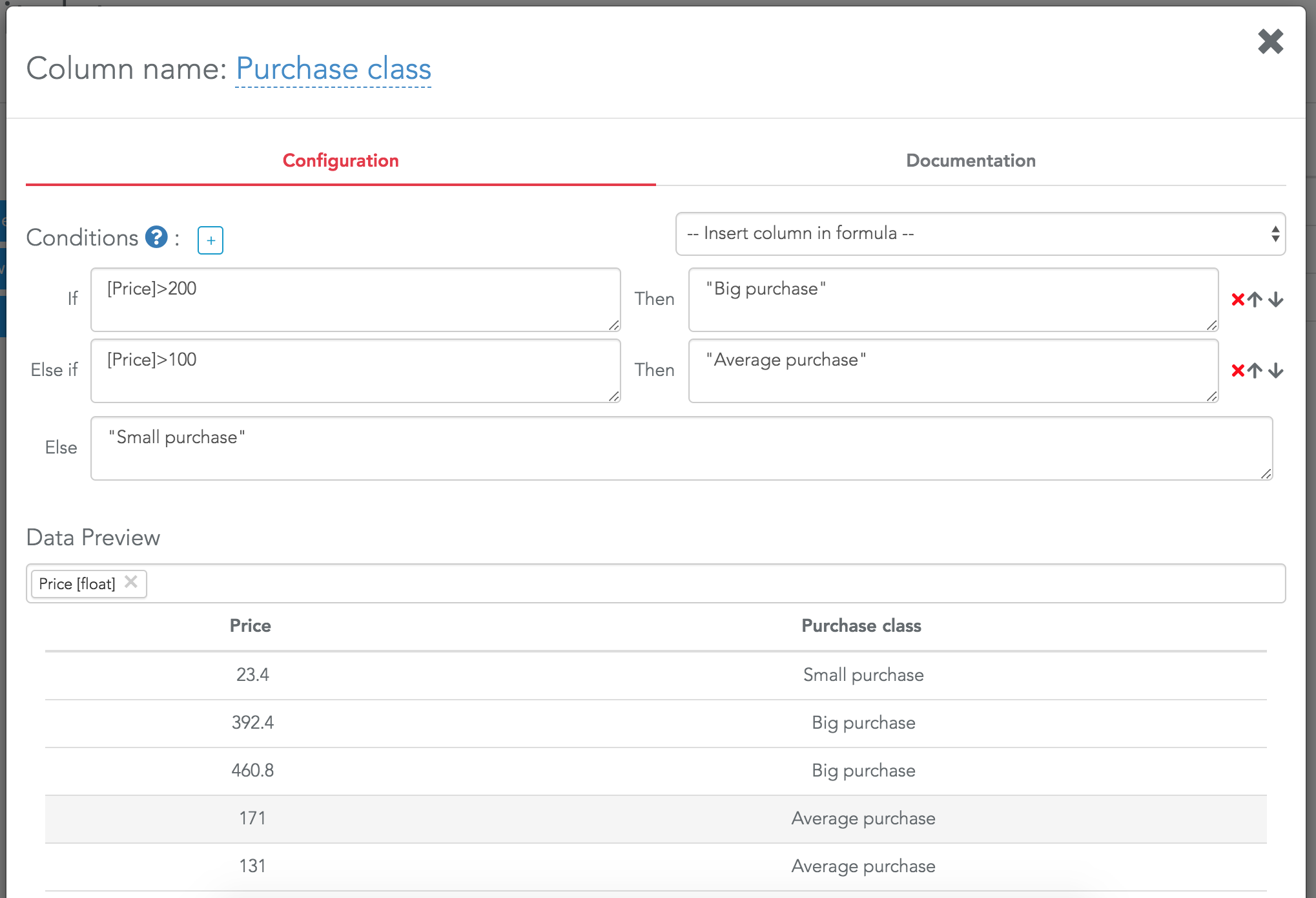Value formula
To add a value formula, click on the "New value formula" button. Then in the formula editor, you must use functions that will return values from columns (e.g. sum, sum_if, dcount...). Here is a simple formula to get the sum of the column Price: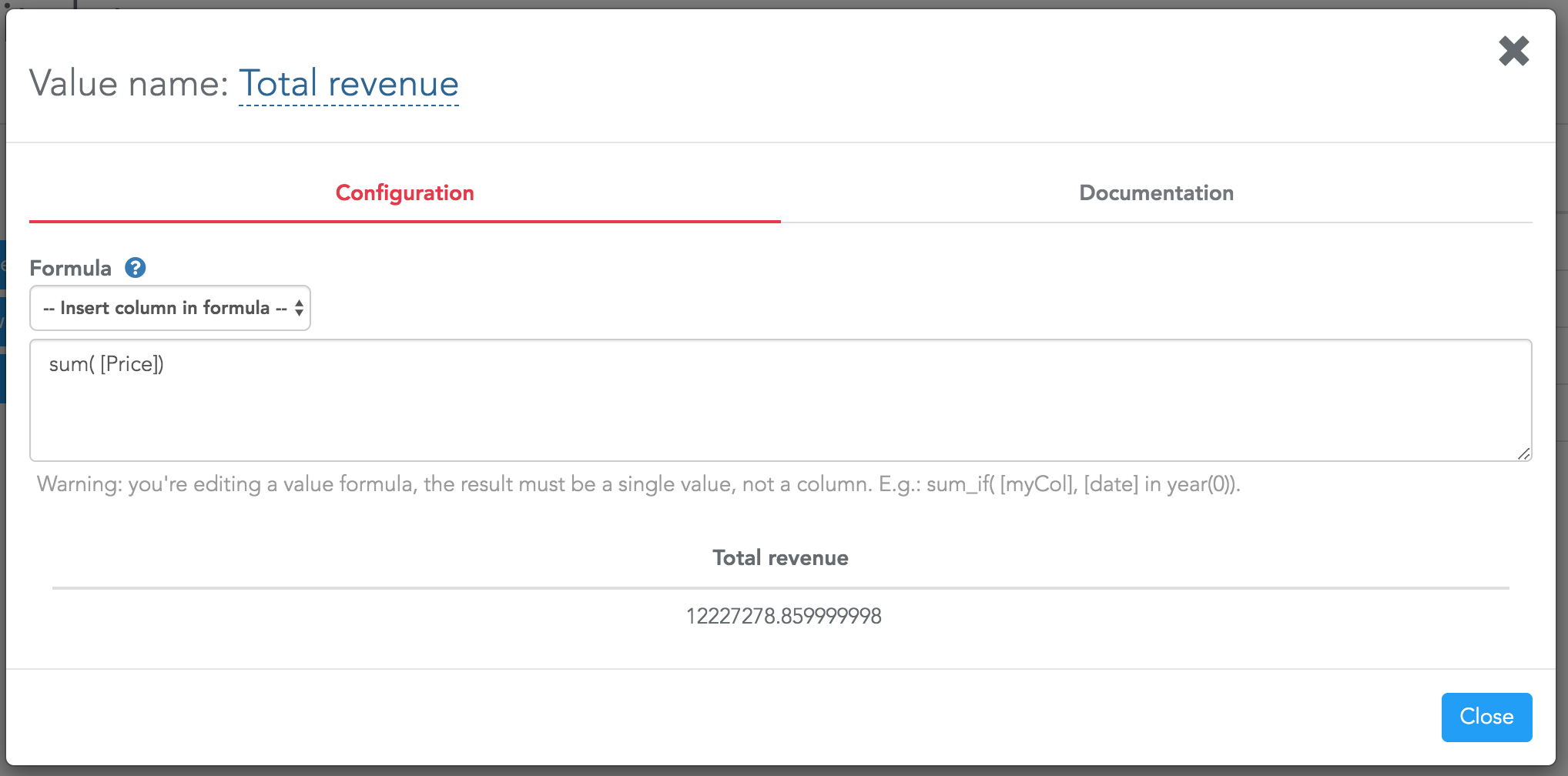You can go further by using several functions and also using the conditional column formula created before: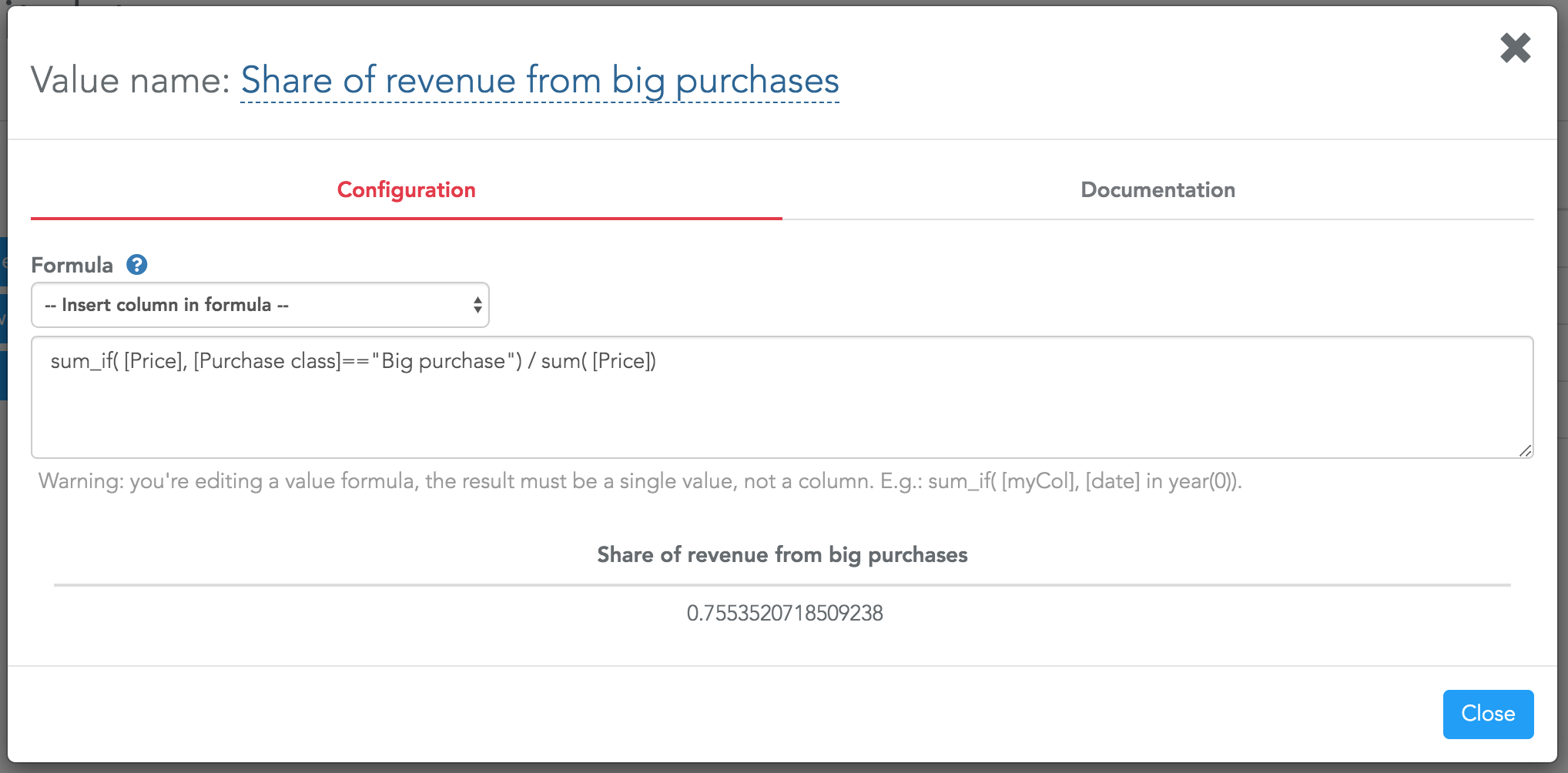Good practices with formulas

From this tutorial and our documentation, you can now create a lot of formulas and kpis.

Try to keep in mind these best practices:
• Give meaningful names to your formulas. For internal projects, we add "fx-" at the beginning of each formula column, and "fx-kpi-" at the beginning of each value formula.
• If the formula is not trivial, write a few words about it in the documentation tab. So when you open your formula 6 months later, you quickly understand why you did it this way.
• Try to create small and easy to understand formulas and to reuse them inside other formulas instead of writing large and complex formulas.
×